#Quality Digest

Featured Product
This Week in Quality Digest Live
Quality Insider Features
Matthew Bundy
Fire protection system design and regulation of flammable materials can be improved with accurate knowledge of fire growth
Zach Winn
Digital tools for responding to a rapidly changing risk landscape
Gleb Tsipursky
A vaccine is on the way, but so is a new strain of the virus
Annette Franz
Give ‘em the pickle!
ASQ
Selected for forward-thinking approach to the member and educational experience, commitment to a positive and inclusive culture
Quality Insider News
An advanced wireless data collection system for acquiring precision measurement data
March 10–11, 2021
Realistic variations in glossiness could aid fine art reproduction and the design of prosthetics
All of the manufacturer’s X-ray sources are supported including rotating target technology and 450kV microfocus source
Smart manufacturing includes recyclability and energy and waste reduction, leading to sustainability and cost savings
Ideal for measuring large components or multiple small components, quickly, easily, and accurately
Measure workpieces from 25 mm to 42.5 mm with a measuring range of ±100 µm and a repeatability of ≤0.1 µm
Contactless gauging system for measuring steel wire for tires, copper wire for electrical cables, CO2 welding wire, and more
Too often process enhancements occur in silos where there is little positive impact on the big pictureBio

Quality Insider

## A Problem With Outlier Tests

### When can you really use Dixon’s test?

Published: Tuesday, September 2, 2014 - 17:26

Outlier tests such as the W-ratio test and Dixon’s outlier test suffer from a problem that can mislead the user. This article will outline the problem and provide guidelines for the appropriate use of these tests.

### Dixon’s outlier test

In 1953, W. J. Dixon proposed a test for detecting outliers that is similar to the W-ratio test given in my columns for June and November of 2012. Since these tests are concerned with the analysis of a fixed and finite data set, we dispense with the time-order sequence and arrange the k values in numerical order. Let Xdenote the smallest value and let Xdenote the largest value, so that the following relationships are satisfied.For Dixon’s outlier test we are concerned with only the first or the last of the differences in this ordered set.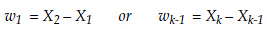Dixon’s Q statistic is the larger of the two differences above divided by the range of the k values (Xk – X1):When the Q statistic exceeds the appropriate critical value then the corresponding extreme value, either X1 or Xk, is said to be an outlier. The critical values for the Q statistic depend upon both the number of values in the original data set, k, and the alpha level for the test. Dixon gave critical values for alpha values ranging from 0.01 to 0.60. Figure 1 contains the critical values for Dixon’s test for alpha levels of 0.01, 0.05, 0.10, and 0.20.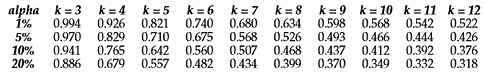Figure 1: Critical values for Dixon’s outlier test

Dixon illustrated this test using the five values { 23.4, 24.1, 25.5, 23.5, 23.2}. Arranging these values in numerical order we have: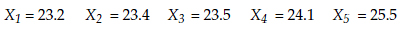so that the first and last differences are: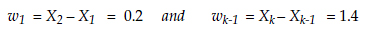and the Q statistic is: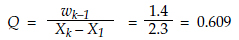Thus, with a 20-percent risk of being wrong, we can label the value of 25.5 to be an outlier in this data set. Dixon’s test is simple, easy to understand, and has been widely used in the 60 years since it was introduced. But there is a problem lurking in the computations that is not widely understood.

### The problem

The critical values given in figure 1 were all obtained under the assumption that the values in the data set are all points from a continuum. That is, the computations assume that each value is known to a large number of decimal places. In practice this is seldom the case. More often than not the data are rounded off to some small number of digits. In the example above, the data were recorded to a tenth of a unit. Thus, while the whole numbers represented measurement units, the measurement increment used was a tenth of a measurement unit.

The fact that all data are recorded to some specific measurement increment can become a problem for outlier tests such as the W-ratio test and Dixon’s Q statistic. To understand this, consider a simple data set consisting of three values that are extremely homogeneous:
X1 = 323.24 lb      X2 = 323.25 lb       X3 = 323.25 lb

The measurement unit here is a pound. The measurement increment is a hundredth of a pound. The Q statistic is:So, with k = 3 and an alpha level of 0.01, Dixon’s test tells us that the value of 323.24 is an outlier! Of course this result makes no sense for these three data.

The problem with using the W-ratio test or Dixon’s Q statistic is that the possible values for the test statistic will depend upon how many measurement increments are contained in the range of the data. In the example above, the smallest and largest values differed by only one measurement increment. As a result, the Q statistic could only take on a value of 0.000 or 1.000. No other values are possible in this case.

In order for both Dixon’s outlier test and the W-ratio test to work as intended, the possible values for the test statistics have to form a reasonable continuum between zero and one. When the range of the data only represents a handful of measurement increments, this condition will not be satisfied, and the alpha-level for the test can be radically different from the nominal alpha level given in the table. For example, if the range of the data set is equal to five measurement increments, then the ratios can only take on the values of 0.0, 0.2, 0.4, 0.6, 0.8, and 1.0, regardless of the value for k.

In Dixon’s example given in the previous section the range was 2.3 units and the measurement increment was one tenth of a measurement unit, so the range corresponds to 23 measurement increments and the Q statistic corresponds to some integer multiple of 1/23 = 0.04348. In this case it was 14 times 1/23 = 0.609. The next possible value for the Q statistic is 0.652. Nothing in between 0.609 and 0.652 is possible.

Thus, while the critical values for the W-ratio test and Dixon’s outlier test assume a continuum of values for the test ratios, in practice we often find the test ratios to be chunky rather than continuous. As a result, the alpha level of our test might be radically different than we think it is, and our analysis may be incorrect. So, when can we use these tests for outliers? This will be addressed in the following section.

### Robust tests for outliers

When we work out the mathematical theory behind a statistical procedure we are usually working with continuous random variables that are independently and identically normally distributed. When we use these same statistical procedures in practice, we are working with data that are recorded to a finite number of digits, are generated by a process that is subject to upsets and changes over time, and never have a normal histogram. As a result, all statistical procedures are approximate. If they work in practice approximately like we expect them to work based on theory, then they are satisfactory. The general term for this satisfactory performance is robustness. Before a statistical test will be of much use it will need to be robust.

We typically characterize robustness by comparing the observed false-alarm rate with the theoretical false-alarm rate. The alpha levels given in figure 1 are these theoretical false-alarm rates. As demonstrated above, the measurement increment can affect the chunkiness of the test ratios, and this in turn can affect the observed alpha level for the procedure.

For example, when the range of data is equal to 10 measurement increments, and there are only k = 3 values in the data, regardless of whether you use the 0.01 critical value, the 0.05 critical value, or the 0.10 critical value, your average observed alpha level is going to be 0.085! Clearly this is a case where the test does not perform as expected based on the mathematical theory. Here Dixon’s test and the W-ratio test are nonrobust.

Thus, the question becomes: How many measurement increments do we need in the range of the data in order for Dixon’s outlier test and the W-ratio test to be robust? The first step in answering this question is to come up with a criterion for robustness. My criterion is as follows: When performing a test using a 0.01 critical value, I expect the observed alpha level to be between 0.0075 and 0.0125; when performing a test using a 0.05 critical value, I expect the observed alpha level to be between 0.040 and 0.060; when performing a test using a 0.10 critical value, I expect the observed alpha level to be between 0.085 and 0.115; and when performing a test using a 0.20 critical value, I expect the observed alpha level to be between 0.17 and 0.23. (The greater latitude given to the 0.01 and 0.05 levels is intentional.) With this criterion your observed alpha level will be reasonably close to the theoretical alpha level that corresponds to the critical value used.

Now, when I use a test for outliers with a single homogeneous data set, it either will, or will not, have a false alarm for that data set. So, to determine the observed alpha level for a test, I will need to repeatedly apply the test to successive homogeneous data sets. If I use Dixon’s test at the 0.01 level with 10,000 homogenous data sets, then according to the criterion given above, I would say the test is operating robustly at the 0.01 level if I find between 75 and 125 false alarms out of the 10,000 tests.

### The simulation study

Since the question is, “How does the number of measurement increments in the range of the data affect the robustness of Dixon’s Q statistic?” we need to look at the observed alpha levels as a function of the number of measurement increments. To compute observed alpha levels I started with several million independent observations from a standard normal distribution. Then I arranged these into data sets of size k (for k = 3 to 10) and computed the Q statistic for each data set. Next I grouped 10,000 sets of size k together and computed an observed alpha level for each of the critical values for that value of k. By repeating this operation up to 25 times for each value of k, I obtained multiple observed alpha levels and an average observed alpha level for each critical value for each value of k. This operation would then be repeated for different numbers of measurement increments in the range of each of the data sets.

For example, when k = 3 and the range of each data set is equal to six measurement increments, regardless of which critical value is used, the average observed alpha level turns out to be 0.145. Even though you may think you are testing at the 1-percent, 5-percent, 10-percent or 20-percent level, you are actually incurring a 14.5-percent risk of a false alarm! Clearly not what the theory predicts.

As the number of measurement increments in the range of each data set gets larger, the effects of the chunky ratios diminish, and eventually the average observed alpha levels will move closer to the theoretical values. Eventually the individual observed alpha levels will also converge on the theoretical values. When all of the individual observed alpha levels for a given critical value meet the criterion for robustness given above, we can say that the test is performing robustly and may be used in practice.

To remove the effects of the simulation study from the results, I started each study by making the range of each set of k data equal to 1,000 measurement increments and adjusting the observed alpha levels to be equal to the theoretical value. Then, as I made the ranges equal to lesser numbers of measurement increments, I could observe the impact these changes had upon the observed alpha levels. As the number of measurement increments got smaller, the variation in the observed alpha levels would increase, and eventually the average alpha levels would start to skew away from the theoretical values. In this way it was possible to discover the minimum number of measurement increments needed in the range of the data set before Dixon’s outlier test can be said to be robust and therefore useful in practice.

The results of these simulation studies are shown in figure 2. There we find the minimum number of measurement increments to be found in the range of the data before we can use Dixon’s outlier test with some hope that our false-alarm rate will be approximately the same as the theoretical alpha level that corresponds to a given critical value.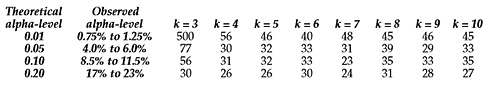Figure 2: Minimum number of measurement increments in range for robustness

If the range of your data set contains fewer measurement increments than the values in figure 2, you cannot reliably use Dixon’s outlier test (or the W-ratio test) at that alpha level. If you do use it, your risk of a false alarm may be considerably different than you think it is. The greater your shortfall in the number of measurement increments, the greater the chance that your alpha level will not be what you think it is.

Notice that Dixon’s example given earlier had only 23 measurement increments in the range, yet from figure 2, with k = 5, we need at least 26 measurement increments to test for outliers at the 20-percent level. So, when Dixon called the value of 25.5 an outlier, he was stretching a point. His alpha level may have been greater than 23 percent, or less than 17 percent due to the chunkiness of his Q statistic.

Except for the first row and the first column of figure 2, most of the values in figure 2 are in the neighborhood of 30. Thus we might generalize to obtain the following guidelines from the simulation study results. If k is 4 or greater and the range of the data consists of at least 30 measurement increments, you may use Dixon’s test at the 0.05, 0.10, or 0.20 levels. If k is 5 or greater and the range of the data consists of at least 45 measurement increments, you may use Dixon’s test at the 0.01 level. In these cases it will be reasonably robust.

To use Dixon’s test for k = 3, you must have a very large number of measurement increments between the minimum and maximum of your three values. Since the expected size of a range drops as the number of values drop, the minimums shown in figure 2 impose a serious barrier to the use of Dixon’s test (or the W-ratio test) with k = 3. (The value of 500 for k = 3 effectively precludes the use of Dixon’s test, or the W-ratio test, in a conservative mode when k = 3.)

### What happens when you find an outlier?

For many years the Imperial Standard Yard (ISY) was the primary standard for length in the United Kingdom. In 1852 they made a secondary standard known as Parlimentary Copy Five (PC5). Of course they compared these two standards periodically. The measurement increment used in these comparisons was a millionth of an inch. The values recorded for [ PC5 – ISY ] in 1852, 1876, 1892, 1912, 1922, and 1932 were, respectively:
{ –55, –33, +70, –43, –23, and –47 millionths of an inch}

Here k = 6, the range of the data is 125 measurement increments, and the Q statistic is 0.744.

Since the data satisfy the requirements for the number of measurement increments in figure 2, and since this Q statistic exceeds the critical values shown for k = 6 in figure 1, we can call the 1892 measurement of +70 an outlier at the 0.01 level. It is clearly different from the other values. Since the standards are presumably not changing, this outlier is most likely due to an error in measurement. (Measuring things to a millionth of an inch is tricky even today. I once watched the readout on a measurement device change by five millionths of an inch whenever I said a word beginning with “p” or “b”.) So, having identified the 1892 reading as an outlier, what can we do? Here we can only delete the 1892 value before computing any summaries. Using the remaining data, PC5 appears to be about 40 millionths of an inch shorter than ISY.

Deleting the outliers may make sense when working with archival data, but in practice, when working with data that supposedly represents your current process and the product that you are actually shipping, this deletion of outliers may be completely misleading. For this reason you should be careful when testing for outliers.

All outliers are signals that something unplanned has happened. When something unplanned happens, you will need to know what has happened. And when you know what has happened you will often need to do something about it. Since unplanned changes in product or process can require action, you would be well-advised to use a conservative (0.01) alpha level when testing for outliers. So, be careful when using a test for outliers—you may regret what you find.

This all means that, in practice, an outlier is usually something much more serious than a blip in the data. It requires something more than simply “cleaning up the data.” If you delete the outlier and go on to compute summary statistics, ship the batches, and approve the process, you will be ignoring the fact that you know things are changing, that the batches are different, and that you have multiple processes masquerading as a single process.

If you choose to do nothing about unplanned changes, if you choose to ignore the outlier as bad data and continue with your computations, then you are simply whistling as you walk past the graveyard. However, in this case, you actually have evidence that there is a zombie in there waiting to eat your brain.

### Discuss### Donald J. Wheeler

Dr. Donald J. Wheeler is a Fellow of both the American Statistical Association and the American Society for Quality, and is the recipient of the 2010 Deming Medal. As the author of 25 books and hundreds of articles, he is one of the leading authorities on statistical process control and applied data analysis. Find out more about Dr. Wheeler’s books at www.spcpress.com.

Dr. Wheeler welcomes your questions. You can contact him at djwheeler@spcpress.com

### Grubbs' test vs. Dixon's test

Dear Sir,

Thank you for sharing such a useful article of outlier test.

Some software use Grubbs's test as one of the options. Which method is more powerful or robust? What is the advantages of using these outlier test since Boxplot could do the job for us?

Regards,

Chee Han LOK